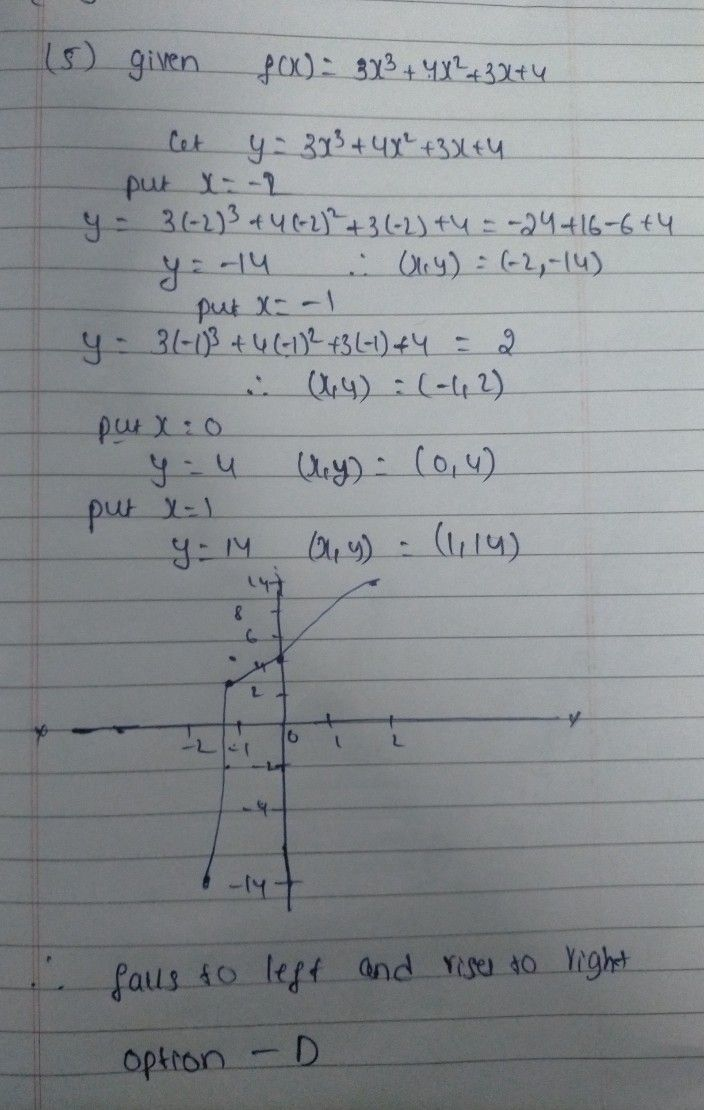Symbol
Problem$5\right)$ $f\left(x\right)=3x^{3}+4x^{2}+3x+4$ A) $\right)$ rises to the left and falls to the right B) rises to the left and rises to the right ) falls to the left and $fa11s$ to the right $D\right)$ falls to the left and rises to the right
10th-13th grade
Algebra
Question content
Use the Leading Coefficient Test to determine the end behavior of the polynomial function.
SolutionQanda teacher - Sandeep